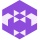Home Communities
IT Knowledge
Inspiration
Languages
EN

# Python - count character occurrences

0 points
Created by:Dale-K1
357

In this article, we would like to show you how to count character occurrences in Python.

Quick solution:

``````text = "ABCBA"
result = text.count("A")

print(result)  # 2``````

## 1. Practical example using string `count()` method

Syntax:

``str.count(substring)``

Where:

• `substring` - Required argument. The string we search for.

Example:

In this example, we use `count()` method to count the number of the "`A`" character occurrences in the `text` string.

``````text = "ABCBA"
result = text.count("A")

print('The number of "A" characters: ', result)  # 2``````

Output:

``The number of "A" characters:  2``

## 2. Practical example using `count()` method with optional arguments

Syntax:

``str.count(substring[, start[, end]])``

Where:

• `start` - Optional argument. The index from which to start counting (by default start of the string, index 0).
• `end` - Optional argument. The end index for the search (by default end of the string).

Example:

In this example, we use the `count()` method with optional arguments to count the number of the "`A`" character occurrences in the `text` string between index `2` and the end of the string (by default).

``````text = 'ABCBA'
result = text.count('A', 2, )

print('The number of "A" characters: ', result)  # 1``````

Output:

``The number of "A" characters:  1``
Join to our subscribers to be up to date with content, news and offers.